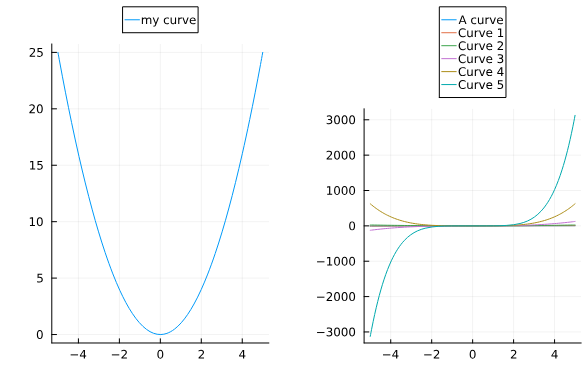# Hot to maintain equal plot sizes of subplot when using e.g. `legend=:outertop`?

MWE:

``````using Plots
p1 = plot(x->x^2, label = "my curve")
plot(x->x^1, label = "A curve")
plot!(x->x^1, label = "Curve 1")
plot!(x->x^2, label = "Curve 2")
plot!(x->x^3, label = "Curve 3")
plot!(x->x^4, label = "Curve 4")
p2 = plot!(x->x^5, label = "Curve 5")

plot(p1, p2, legend=:outertop)
``````Here, I would like the two plots to display of equal size (they use the same axises and should be compared). When the legend is on the outside, this squeezes the plots differently